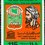# The Curious 6

6 is such a very intriguing number. It is perhaps best known for being the first perfect number, which was noted by Euclid in 300 BCE. A perfect number is a number whose factors sum to the number itself. In the case of 6, $1+2+3=6$ . Perfect numbers are rare beasts indeed; the fact the the 6th perfect number is 8,589,869,056 should demonstrate that!

6 is also a triangle number, which means we can arrange a set of 6 objects into a triangle. It is also the first composite number without repeated prime factors, $6=2 \cot 3$.

Triangle numbers are generated by:

$T_{n} = \frac{(n)(n+1)}{2}$ explicitly or by $T_{n} = T_{n-1} + n$ recursively.

So $T_{3} = 6$, that is, 6 is the triangle number with generator 3. What is the triangle number with generator 6? Well,

$T_{6} = \frac{(6)(6+1)}{2} = \frac{56}{2} = 28$.

This may seem unremarkable, until we realize 28 is the next consecutive perfect number after 6! Adding up the factors of 28:

$T_{6} = 28 = 1 + 2 + 4 + 7 + 14$.

I do not know if 6 being the triangle generator of 28 is related to them both being perfect. All known perfect numbers are also triangle numbers, but this does not mean they generate one another. For instance, the next perfect number after 28 is 496, which is $T_{31}$ not $T_{28}$. It is only 6 that manages to do both.

There is one other curious property of 6 that had me thinking, and perhaps offers insight into relating these things more elegantly. 6 is the first number base with a number less than itself (4) that does not divide into the base itself but does divide perfectly into a power of the base (in this case $6^{2} = 36$ and $4 | 36$ ). The | symbol there means "divides into", but is not used much outside of modular arithmetic. Thus, 4 is neither coprime with 6, nor a divisor of it. If nothing else, it indicates that 36 has a lot of factors!

I'm wondering if any fellow 6-lovers have any more insight on this.Note by Alex Munger
1 year, 5 months ago

This discussion board is a place to discuss our Daily Challenges and the math and science related to those challenges. Explanations are more than just a solution — they should explain the steps and thinking strategies that you used to obtain the solution. Comments should further the discussion of math and science.

When posting on Brilliant:

• Use the emojis to react to an explanation, whether you're congratulating a job well done , or just really confused .
• Ask specific questions about the challenge or the steps in somebody's explanation. Well-posed questions can add a lot to the discussion, but posting "I don't understand!" doesn't help anyone.
• Try to contribute something new to the discussion, whether it is an extension, generalization or other idea related to the challenge.
• Stay on topic — we're all here to learn more about math and science, not to hear about your favorite get-rich-quick scheme or current world events.

MarkdownAppears as
*italics* or _italics_ italics
**bold** or __bold__ bold
- bulleted- list
• bulleted
• list
1. numbered2. list
1. numbered
2. list
Note: you must add a full line of space before and after lists for them to show up correctly
paragraph 1paragraph 2

paragraph 1

paragraph 2

[example link](https://brilliant.org)example link
> This is a quote
This is a quote
    # I indented these lines
# 4 spaces, and now they show
# up as a code block.

print "hello world"
# I indented these lines
# 4 spaces, and now they show
# up as a code block.

print "hello world"
MathAppears as
Remember to wrap math in $$ ... $$ or $ ... $ to ensure proper formatting.
2 \times 3 $2 \times 3$
2^{34} $2^{34}$
a_{i-1} $a_{i-1}$
\frac{2}{3} $\frac{2}{3}$
\sqrt{2} $\sqrt{2}$
\sum_{i=1}^3 $\sum_{i=1}^3$
\sin \theta $\sin \theta$
\boxed{123} $\boxed{123}$

## Comments

There are no comments in this discussion.

×

Problem Loading...

Note Loading...

Set Loading...